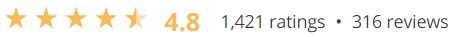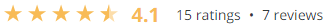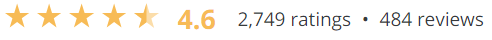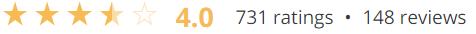Showing all 5 results

Show sidebar

### Introduction to Mathematical Thinking

\$49.00Learn how to think the way mathematicians do - a powerful cognitive process developed over thousands of years. read more...

### Mathematics for Computer Science

\$49.00Welcome to Introduction to Numerical Mathematics. This is designed to give you part of the mathematical foundations needed to work in computer science in any of its strands, from business to visual digital arts, music, games. read more...

### Mathematics for Machine Learning: Linear Algebra

\$49.00In this course on Linear Algebra we look at what linear algebra is and how it relates to vectors and matrices. Then we look through what vectors and matrices are and how to work with them, including the knotty problem of eigenvalues and eigenvectors, and how to use these to solve problems. read more...

### Mathematics for Machine Learning: Multivariate Calculus

\$49.00This course offers a brief introduction to the multivariate calculus required to build many common machine learning techniques. We start at the very beginning with a refresher on the "rise over run" formulation of a slope, before converting this to the formal definition of the gradient of a function. read more...

### Mathematics for Machine Learning: PCA

\$69.00This intermediate-level course introduces the mathematical foundations to derive Principal Component Analysis (PCA), a fundamental dimensionality reduction technique. read more...